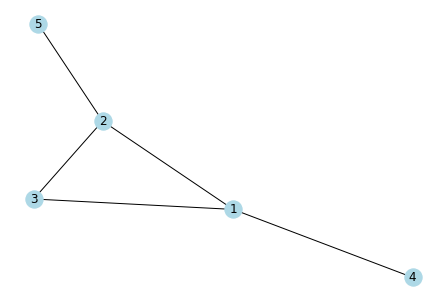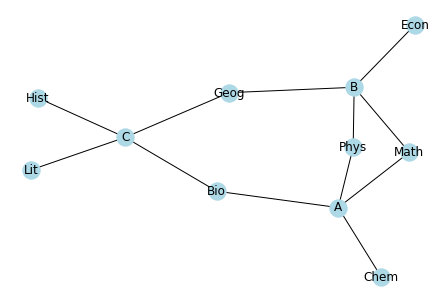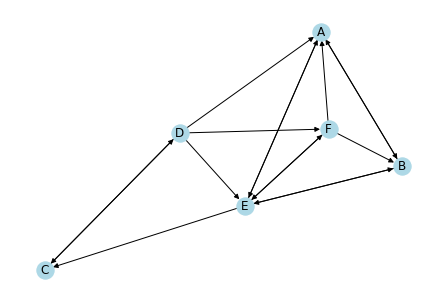# Networks¶

In this exercise, you will use the Python library Networkx to analyze different graphs and networks.

Here's a quick recap of how to compute the eigenvalues and eigenvectors of the adjacency matrix of a graph.

In :
import numpy as np
import networkx as nx

# Undirected graph
G = nx.Graph()
edges = [(1,2),(1,3),(1,4),(2,3),(3,2),(4,4),(5,2)]

# Draw the graph
nx.draw(G, with_labels = True, node_color = 'lightblue')Getting the adjacency matrix of the graph:

In :
A = nx.adj_matrix(G).todense()
A

Out:
matrix([[0, 1, 1, 1, 0],
[1, 0, 1, 0, 1],
[1, 1, 0, 0, 0],
[1, 0, 0, 1, 0],
[0, 1, 0, 0, 0]], dtype=int32)

Getting the eigenvalues and eigenvectors of A

In :
d, E = np.linalg.eig(A)
d, E

Out:
(array([ 2.4038748 , -1.54301627, -1.21033197,  0.19253139,  1.15694205]),
matrix([[-0.56938065, -0.49286086,  0.59874578,  0.24242708, -0.12500581],
[-0.51291304,  0.70263065,  0.23535076, -0.14728067,  0.40761354],
[-0.45022881, -0.13594788, -0.68914692,  0.49418646,  0.2442713 ],
[-0.40557794,  0.19380956, -0.270885  , -0.30023096, -0.79650931],
[-0.21336928, -0.45536179, -0.19445141, -0.76496969,  0.35231975]]))

Accessing a particular entry of d (recall that indexing starts from 0):

In :
d

Out:
-1.21033196569278

Accessing the corresponding column of E:

In :
E[:,2]

Out:
matrix([[ 0.59874578],
[ 0.23535076],
[-0.68914692],
[-0.270885  ],
[-0.19445141]])

# Homework¶

Choose one of the following two questions to do. You only have to submit answers for the "Code this" and "Answer this" portion, but I hope you also try the "Think about this first" part.

You can submit your answers to the "Answer this" part as comments in your code. Or you can write it out or type it in the email if you prefer.

## Question 1: Bipartite graphs¶

Copy the following code to define a bi-partite graph B. (You don't have to understand everything in the code, but feel free to ask me if you would like to know what's being done).

In :
import numpy as np
import networkx as nx

# Data
students = ['A', 'B', 'C']
subjects = ['Phys', 'Chem', 'Bio', 'Math', 'Hist', 'Lit', 'Geog', 'Econ']
student_subjects = {
'A': ['Phys','Chem', 'Bio', 'Math'],
'B': ['Math', 'Econ', 'Geog','Phys'],
'C': ['Hist', 'Lit', 'Geog', 'Bio']
}

# Empty (undirected) graph
B = nx.Graph()

# Add nodes (this is will determine the order of the rows and columns of the adjacency matrix)

for student in students:
for subject in student_subjects[student]:

In :
print(B.nodes)
nx.draw(B, with_labels = True, node_color = 'lightblue')

['A', 'B', 'C', 'Phys', 'Chem', 'Bio', 'Math', 'Hist', 'Lit', 'Geog', 'Econ']Think about this first: From the drawing of B, it's not very obvious that it is bi-partite. Can you think of a way to determine whether a given graph is bipartite?

Code this: Now use what you've learned to get the eigenvalues and eigenvectors of (the adjacency matrix of) B.

Answer this: Look at the eigenvector corresponding to the smallest (i.e. most negative) eigenvalue, and see which entries are assigned to which nodes (the entries are arranged according to B.nodes). How can you use this eigenvector to divide a bipartite graph into its two parts?

## Question 2: Ranking graphs¶

Copy the following code to define a directed graph G. (You don't have to understand everything in the code, but feel free to ask me if you would like to know what's being done).

In :
import numpy as np
import networkx as nx

# Data
teams = ['A', 'B', 'C', 'D', 'E', 'F']
matches_won = {
'A' : ['B', 'E'], # This means team A beat teams B and E
'B' : ['A', 'E'],
'C' : ['D'],
'D' : ['A', 'C', 'E', 'F'],
'E' : ['A', 'B', 'C', 'F'],
'F' : ['A', 'B', 'E']
}

# Empty directed graph
G = nx.DiGraph()

# Add nodes (this is will determine the order of the rows and columns of the adjacency matrix)

for winner in teams:
for loser in matches_won[winner]:

In :
print(G.nodes)
nx.draw(G, with_labels = True, node_color = 'lightblue')

['A', 'B', 'C', 'D', 'E', 'F']Think about this first: This graph represents the results of an informal tournament, where teams need not play the same number of games. There is a directed arrow from X to Y if X beat Y in a match. Looking at the graph (or at matches_won in the code defining the graph), which would you say is the strongest team? The weakest team?

Code this: Now use what you've learned to get the eigenvalues and eigenvectors of (the adjacency matrix of) G.

Answer this: Look at the eigenvector corresponding to the largest eigenvalue, and see which entries are assigned to which nodes (the entries are arranged according to G.nodes). How can you use this eigenvector to rank teams?